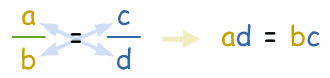# 交叉相乘

 交叉相乘是从这个： 8 12 = 2 3 变成这个： 8 × 3 = 12 × 2

## 怎样运算？

8 × 3 12 × 3 = 2 3

8 × 3 12 × 3 = 2 × 12 3 × 12

8 × 3 = 12 × 2

## 用变量

 交叉相乘是从这个： a b = c d 变成这个： ad = bc### 例子：求 "x"：

 x 8 = 2 x 交叉相乘： x2 = 8 × 2 计算： x2 = 16 解： x = 4 或 −4

## 词汇